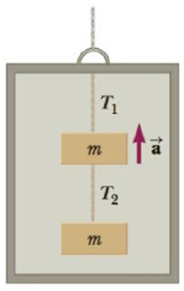Chapter 4, Problem 71P

Chapter
Section
Textbook Problem

Two blocks each of mass m = 3.50 kg are fastened to the top of an elevator as in Figure P4.56. (a) If the elevator has an upward acceleration a = 1.60 m/s2, find the tensions T1 and T2 in the upper and lower strings. (b) If the strings can withstand a maximum tension of 85.0 N, what maximum acceleration can the elevator have before the upper string breaks?(a)

To determine
The tension in the strings.

Explanation

Given info: The mass of the two blocks is 3.50 kg. Acceleration of the elevator is 1.60ms2 .

The free body diagrams are given below.

From the diagrams,

T2mg=ma (I)

T1T2mg=ma (II)

• T1 and T2 are the tensions on the upper and lower strings.
• m is the mass of the blocks.
• g is the acceleration due to gravity.
• a is the acceleration of the elevator.

On Re-arranging Equation (I),

T2=m(a+g)

Substitute 3.50 kg for m, 9.8ms2 for g and 1.60ms2 for a to get T2 .

T2=(3.50kg)(1.60ms2+9

(b)

To determine
The maximum acceleration before the upper string breaks.

Still sussing out bartleby?

Check out a sample textbook solution.

See a sample solution

The Solution to Your Study Problems

Bartleby provides explanations to thousands of textbook problems written by our experts, many with advanced degrees!

Get Started

What is a standard unit?

An Introduction to Physical Science

What are the symptoms of vitamin B12 deficiency?

Introduction to General, Organic and Biochemistry

Describe how bone first develops.

Human Biology (MindTap Course List)